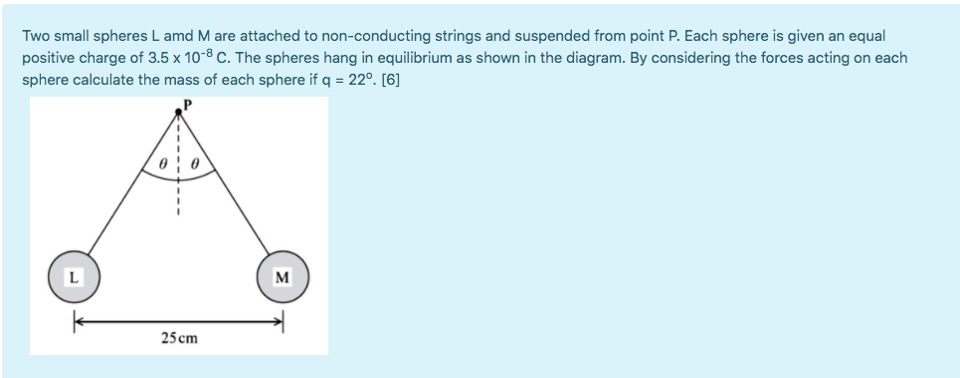# Two small spheres L amd M are attached to non-conducting strings and suspended from point P. Each sphere is given an equal positive charge of 3.5 x 10-8 C. The spheres hang in equilibrium as shown in the diagram. By considering the forces acting on ead sphere calculate the mass of each sphere if q = 22°.  L M 25 cm

Question

Ghelp_outlineImage TranscriptioncloseTwo small spheres L amd M are attached to non-conducting strings and suspended from point P. Each sphere is given an equal positive charge of 3.5 x 10-8 C. The spheres hang in equilibrium as shown in the diagram. By considering the forces acting on ead sphere calculate the mass of each sphere if q = 22°.  L M 25 cm fullscreen

### Want to see this answer and more?

Experts are waiting 24/7 to provide step-by-step solutions in as fast as 30 minutes!*

*Response times may vary by subject and question complexity. Median response time is 34 minutes for paid subscribers and may be longer for promotional offers.
Tagged in
Science